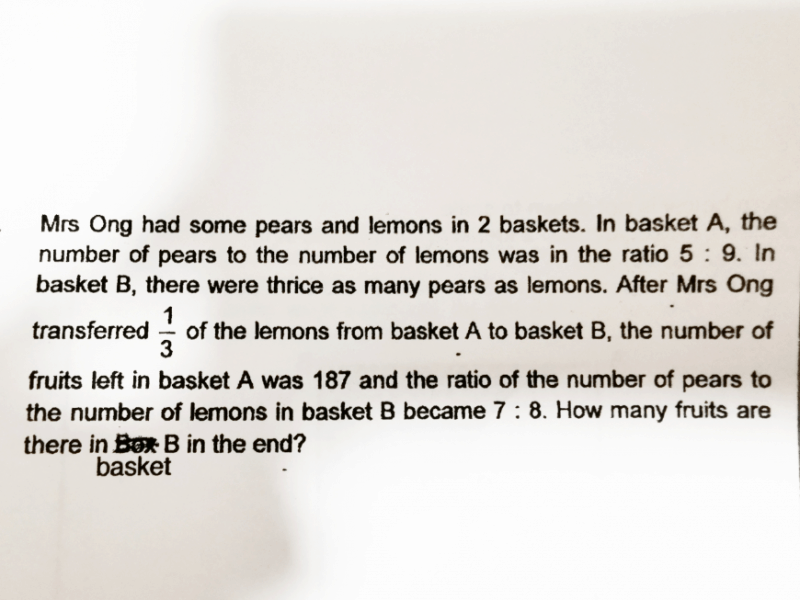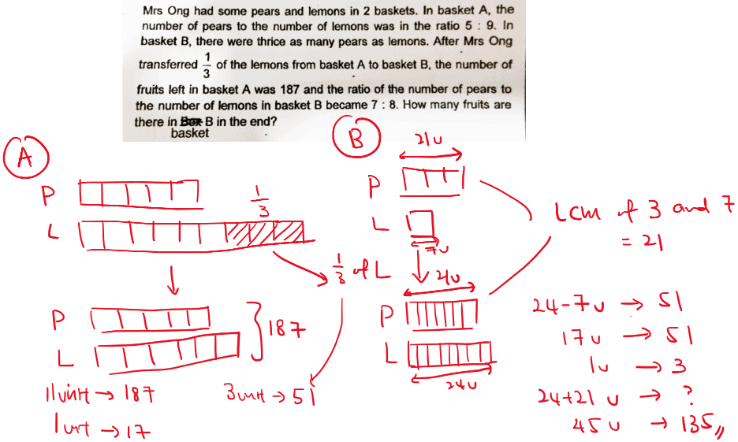# QuestionHi

Can someone help to explain the above question?

Thank you.This is a Before and After Concept where students need to draw some models in order to comprehend and solve the problem.

First concept is that student needs to know that out of the 9 units of lemon in A, 3 are transferred and you are left with 6 units. After which you can calculate how many lemons are transferred.

The next concept is that the number of pears in B remains unchanged and you can make use of common multiple to find the common number of units for pear and equate the difference to the number of lemons transferred.

These two are the key concepts your child needs to know to do such questions. Do guide your child in understanding these two concepts.

At Enso Education, we focus on understanding such concepts through model drawing which is simple to draw and comprehend.

www.ensoeducate.com/sgcourses